# How Formulas Work

A formula is a cell entry that calculates values to return a result. Each Excel formula must have three key elements: the equal sign ( = ) that signifies that the entry is a formula, the values or cell references to be calculated, and the mathematical operators, such as a plus sign ( + ) for addition or a minus sign ( - ) for subtraction.

All Excel formulas must begin with the equal sign. The equal sign tells Excel that the entry is a formula. If your formula begins without an equal sign, Excel treats it as a regular cell entry and doesn't perform the calculation.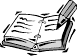If you begin a formula with a plus sign ( + ), Excel converts it to an equal sign ( = ). That's because Lotus 1-2-3, another spreadsheet program, uses the plus sign as its opening formula-entry character. Excel makes it easy for Lotus users to switch to Excel.

#### Mathematical Operators

When you create a formula in Excel, you need to include an operator. All formulas must contain mathematical operators so that Excel knows what calculation to perform. Table 44.1 lists the arithmetic operators used in Excel.

##### Table 44.1. Excel Formula Operators

Operator

What It Does

+

-

Subtraction

*

Multiplication

/

Division

=

Equal to

>

Greater than

>=

Greater than or equal to

<>

Not equal to

%

Percentage

^

Exponentiation

Figure 44.1 shows a very simple formula. The intent is pretty clear; the formula asks Excel to multiply 8 by 47 .

##### Figure 44.1. The formula in cell A1 contains a mathematical operator.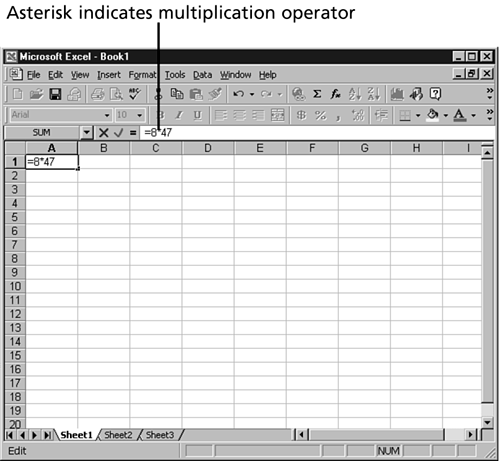#### Order of Operations

In a simple formula, such as the one shown in Figure 44.1, Excel is asked to perform only one calculation. However, formulas can often contain instructions to perform multiple calculations. If you were to talk out a more complicated formula, you might say something like this: Add together the price of a toy truck that costs \$18.75 and a doll that costs \$13.00 and then multiply the combined price by 7.5% sales tax to determine the sales tax due on the combined cost of the toys.

You might think that you'd enter the value for the formula this way:

` =18.75+13.00*.075 `

However, if you typed that formula in a cell, Excel would produce the wrong answer. The reason is that Excel uses something called operator precedence to perform calculations. Operator precedence determines the order in which calculations are performed. Calculations are performed from left to right in the following order:

1. All operations enclosed in parenthesis

2. All exponential operations

3. All multiplication and division operations

4. All addition and subtraction operations

The best way to force Excel to calculate your formulas correctly is to use parentheses. Group the values and operators that you want to calculate first in parentheses. For example, the formula

` (18.75+13.00)*.075 `

tells Excel to first add the numbers within the parentheses and then to calculate the sales tax percentage on the total.

You can even nest parentheses within parentheses to further break down how you want Excel to calculate your formula. Just remember that each opening parenthesis must have a closing parenthesis. If your formula does not contain the required number of parentheses, Excel displays an error message similar to the one in Figure 44.2. Excel even attempts to place the missing parenthesis. If your formula doesn't contain the proper number of parentheses, Excel displays each parentheses set in a different color , to help you track your error.

##### Figure 44.2. Excel tells you when a formula has not been entered correctly.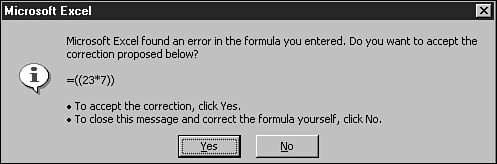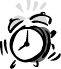When Excel detects a formula error due to too many or too few parenthesis, it attempts to place the missing characters . If you let Excel correct your formula, check it over carefully . After all, Excel doesn't know what your calculation should accomplish.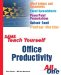Sams Teach Yourself Office Productivity All in One (Sams Teach Yourself All in One)
ISBN: 0672325349
EAN: 2147483647
Year: 2003
Pages: 474
Authors: Greg Perry